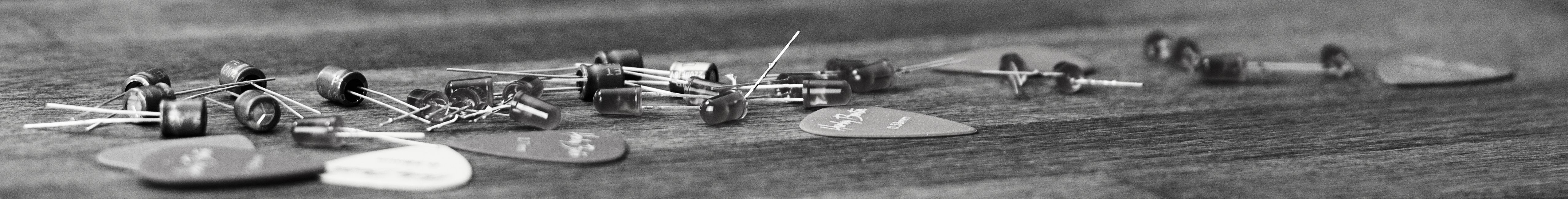savchenko.tech / software engineering blog by Maryna Savchenko2022-09-10

A Linked List is fundamental and incredibly useful. It enables implementation of bags, queues and stacks.

It is a recursive data structure. In the core of Linked List is Node that contains of generic item and reference to the next node.Node abstraction would be a nested class in Linked List and would look like this:

``````private static class Node<E> {
E value;
Node<E> next;

public Node(E value) {
this.value = value;
}
}
``````

Insertion an element to the beginning of the Linked List.

To check that insertion is working correctly we need next test cases:

• size of the list should increase correctly
``````    @Test

}
``````

To add value to the beginning of the list we need to simply point the next link of the new node to the head and assign head to the new node.

``````public void add(E value) {
Node<E> newNode = new Node<>(value);
size++;
}
``````

Remove an element from the beginning of the Linked list.

To remove the value from the beginning of the list we need to simply assign head to the next element.

``````public E removeFromHead() {
size--;
return first.value;
}
``````

In test cases we need to check that head is pointing to the first added element. Because insertion is happening to the beginning of the list (LIFO).

``````    @Test

}
``````

To reverse Linked list we need to maintain references to 3 consecutive nodes, first, second and reversed. At each iteration we extract the node from the original list (first) and add it to the beginning of the reversed list. Second node is the second node of what is left of the original list and Reversed is the head of reversed list.

``````public Node<E> reverseList() {
Node<E> reversed = null;
while (first != null) {
Node<E> next = first.next;
first.next = reversed;
reversed = first;
first = next;
}